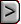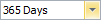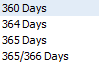﻿ What is the Basis Year
WinAmort Professional 2.0

What is the Basis YearWhat is the Basis YearWhat is the Basis YearPress

The Basis Year option, will allow you to set the length of year. To change the year length:Down Arrow button beside the Basis Year field.

A list of different days in the year will appear.Choose 360 to compute daily interest for odd periods using 1/360 of the nominal annual rate.  This method is useful when you are working with monthly compounding and want to treat odd days as 1/30 of a month.   Choose 364 to compute daily interest for odd periods using 1/364 of the nominal annual rate.  This method is useful when you are working with weekly payments and want your daily rate for stub periods to be exactly 1/7 of the weekly rate.   Choose 365 to compute daily interest for odd periods using 1/365 of the nominal annual rate.

Select the number of days that you want to set the year length using the mouse.

The year length is used to establish the daily rate of interest for odd days.

If a \$100,000 loan at 12% interest is taken out on 11/05/1997, and the payments are to start on 12/15/1997, there would be 10 odd days from the 5th of November to 15th of December. The interest for the odd days would be as follows:

For 360 day Year: 10 * (.12/360) * \$100000 = \$333.33

For 364 day Year: 10 * (.12/364) * \$100000 = \$329.67

For 365 day Year: 10 * (.12/365) * \$100000 = \$328.77

If the dates were 11/05/2010 and 11/15/2010 for loan and payment dates respectively, the odd days interest would still be the same as above because there are 10 odd days.

In most situations, the Basis Year setting will not be critical. But, when dealing with calculations that involve odds days, or when the compounding frequency is set to Daily, the Basis Year setting becomes very significant.

365    Choose 365 to compute daily interest for odd periods using 1/365 of the nominal annual rate.

364    Choose 364 to compute daily interest for odd periods using 1/364 of the nominal annual rate.  This method is useful when you are working with weekly payments and want your daily rate for stub periods to be exactly 1/7 of the weekly rate.

360    Choose 360 to compute daily interest for odd periods using 1/360 of the nominal annual rate.  This method is useful when you are working with monthly compounding and want to treat odd days as 1/30 of a month.